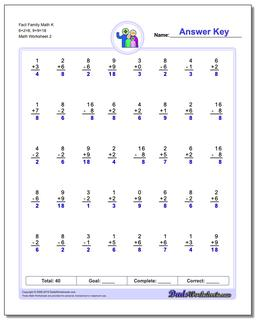One Dad. Four daughters. 9,251 worksheets... and counting!# Fact Family Math K 6+2=8, 9+9=18 (Second Worksheet)

PropertyValue
DescriptionFact Family Math K 6+2=8, 9+9=18: These 40 problem addition and subtraction fact family worksheets are perfect for either quick practice or a one-minute timed test. Be sure to check out the stand-alone addition worksheets and subtraction worksheets for help on specific operations. (Second Worksheet)
Resource TypeWorksheet